#Function Repository Resource:

# SimplexOrientation

Get the orientation of a simplex

Contributed by: Richard Hennigan (Wolfram Research)
 ResourceFunction["SimplexOrientation"][simplex] returns the orientation of simplex. ResourceFunction["SimplexOrientation"][{simplex1,simplex2,…}] returns the orientation of simplices in the given complex.

## Details and Options

A simplex can be specified by any of the following:
 Point[v] a point Line[{v1,v2}] a line segment Triangle[{v1,v2,v3}] or Polygon[{v1,v2,v3}] a filled triangle Tetrahedron[{v1,v2,v3,v4}] a filled tetrahedron Simplex[{v1,v2,…,vn}] an n-1 dimensional simplex

## Examples

### Basic Examples

Get the orientation of a simplex:

 In:=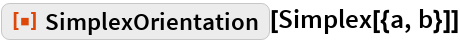Out=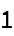In:=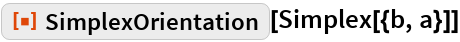Out=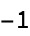Find the permutations of the vertices of a simplex that are equivalent to the original simplex:

 In:=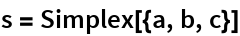Out=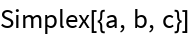In:=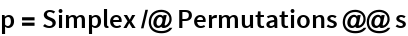Out=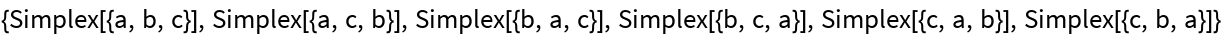In:=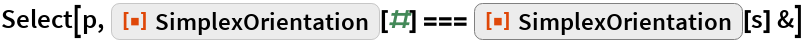Out=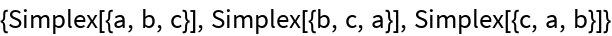Get orientations for a list of simplices:

 In:=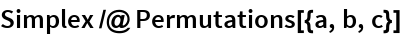Out=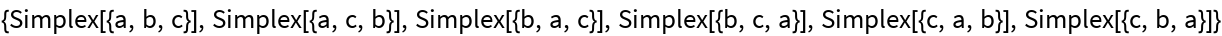In:=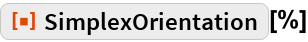Out=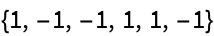### Scope

SimplexOrientation works with Point:

 In:=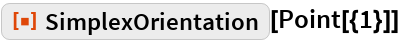Out=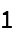SimplexOrientation works with Line:

 In:=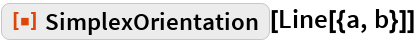Out=In:=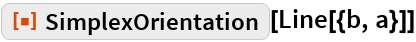Out=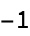SimplexOrientation works with Triangle:

 In:=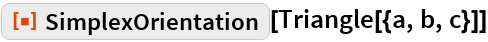Out=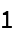In:=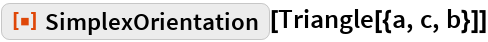Out=SimplexOrientation works with Tetrahedron:

 In:=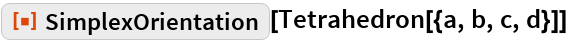Out=In:=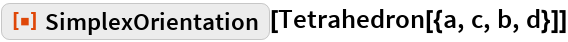Out=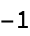Visualize orientation using Graphics3D and FaceForm:

 In:=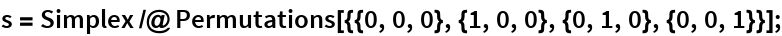In:=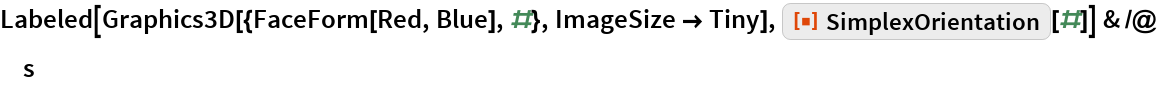Out=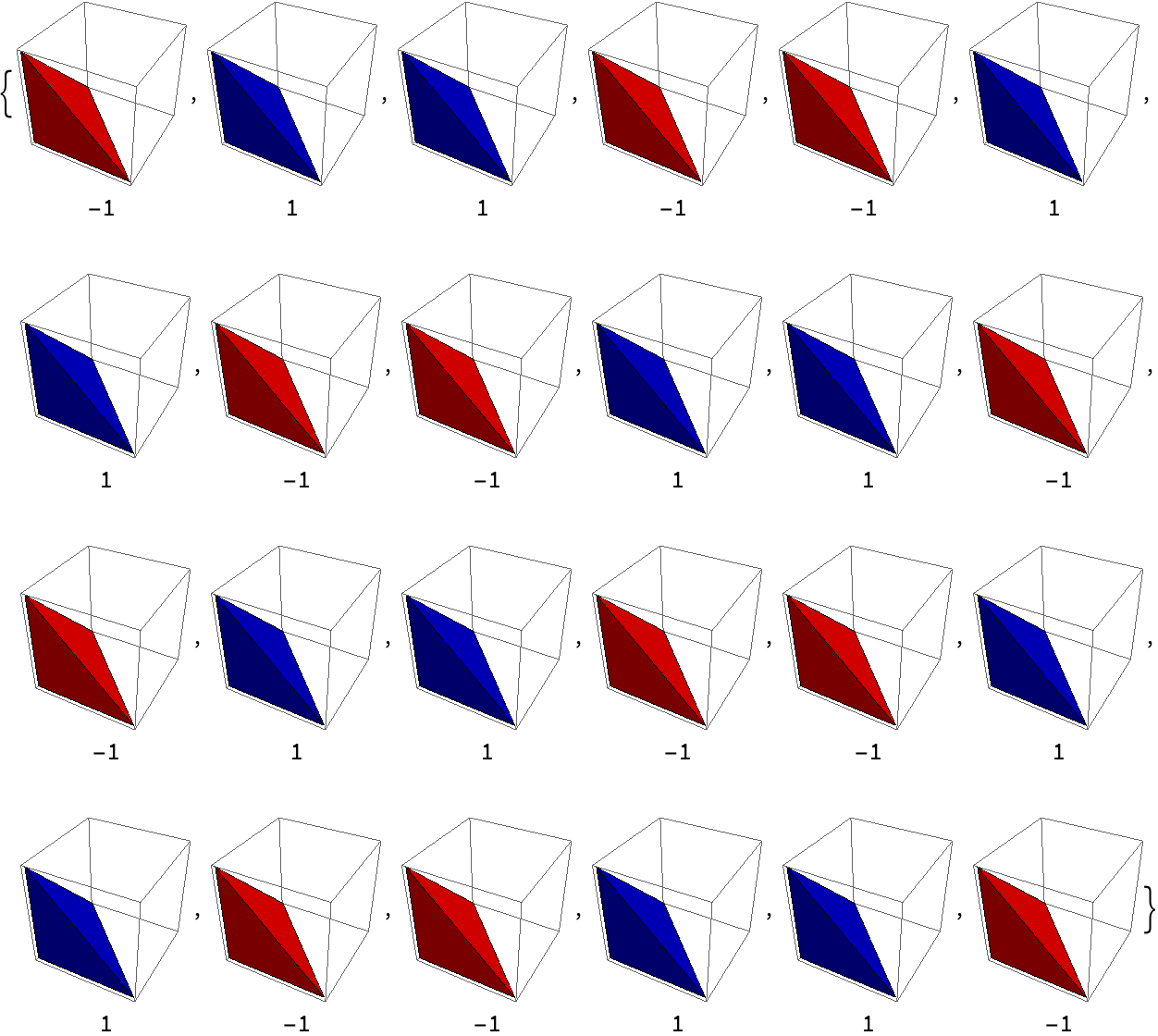Simplices can have arbitrary expressions as vertices:

 In:=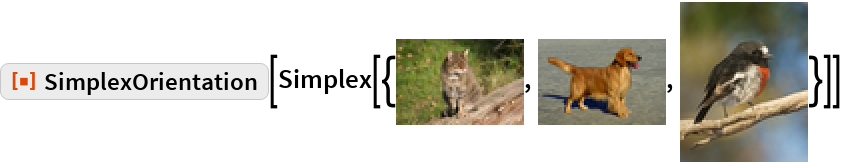Out=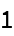We can see that the cat-dog-bird simplex has the opposite orientation to the cat-bird-dog simplex:

 In:=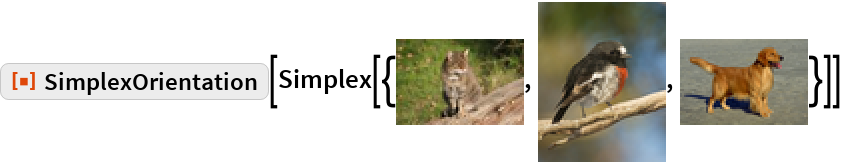Out=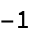### Properties and Relations

Check the output of the three argument form of StandardSimplex:

 In:=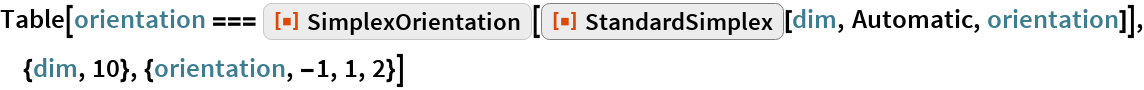Out=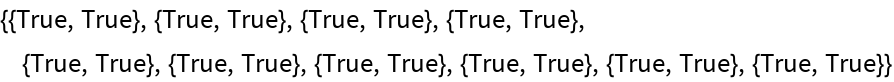## Requirements

Wolfram Language 11.3 (March 2018) or above